Electricity - NCERT Questions
Q 1.

What does an electric circuit mean?

SOLUTION:

A continuous conducting path consisting of some electric components connected between the two terminals of a battery is called an electric circuit.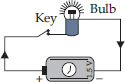An electric circuit generally consisting of a bulb (1.5 V), a key, copper wires and a dry cell as shown in figure.

Q 2.

Define the unit of current.

SOLUTION:

Unit of current is ampere (A). When 1 coulomb of charge flows through a conductor in 1 second, then the current flowing through the conductor is called
1 ampere.

Q 3.

Calculate the number of electrons constituting one coulomb of charge.

SOLUTION:

Charge, Q = 1 C
Charge on an electron, q = 1.6 × 10–19 C
No. of electrons constituting 1 C of charge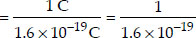= 6.25 × 1018 electrons

Q 4.

Name a device that helps to maintain a potential difference across a conductor.

SOLUTION:

A battery consisting of one or more electric cells is used to maintain a potential difference across a conductor.

Q 5.

What is meant by saying that the potential difference between two points is 1 V?

SOLUTION:

Potential difference between two points in a current-carrying conductor is said to be 1 volt if 1 joule of work is done to carry a charge of 1 coulomb from one point to the other, i.e.,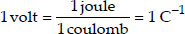Q 6.

How much energy is given to each coulomb of charge passing through a 6 V battery?

SOLUTION:

Given: Charge, Q = 1 C
Applied voltage, V = 6 V
Then,
Work done in moving a charge of 1 C under the voltage of 6 V = 1 C × 6 V = 6 J Thus, an energy of 6 J should be given during the passage of one coulomb of charge through a 6 V battery.

Q 7.

On what factors does the resistance of a conductor depend?

SOLUTION:

A solution having same composition throughout is homogeneous.

Q 8.

Will current flow more easily through a thick wire or a thin wire of the same material when connected to the same source? Why?

SOLUTION:

The current flows more easily through a thick wire than through a thin wire. This is due to the reason that the resistance R of a thick wire of large area of cross-section, A is less than that of a thin wire of small A as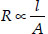Q 9.

Let the resistance of an electrical component remain constant while the potential difference across the two ends of the component decreases to half its former value. What change will occur in the current through it?

SOLUTION:

When the potential difference across the two ends of the electrical component becomes half its former value, the current through it also becomes half. Since I = V/R, when potential difference becomes V/2, current becomes I/2 as the resistance (R) of the component remains constant.

Q 10.

Why are the coils of electric toasters and electric irons made of an alloy rather than a pure metal?

SOLUTION:

Alloys are used for making the coils in electric toaster and electric iron because alloys have higher melting point than pure metals. So, the coils made from alloys do not melt or get deformed and alloys do not get oxidised or burn readily at high temperatures.

Q 11.

Use the data in Table 1(12.2 in NCERT) to answer the following :
(A) Which among iron and mercury is a better conductor?
(B) Which material is the best conductor?

SOLUTION:

(A) Iron is a better conductor than mercury as resistivity (ρ) for iron
(10.0 × 10–8 Ω m) is less than that for mercury
(94 × 10–8 Ω m).
(B) Silver is the best conductor as its resistivity (ρ) is the least, i.e., 1.60 × 10–8 Ω m

Q 12.

Draw a schematic diagram of a circuit consisting of a battery of three cells of 2 V each, a 5 Ω resistor, a 8 Ω resistor, and a 12 Ω resistor, and a plug key, all connected in series.

SOLUTION:

The schematic circuit diagram is shown below: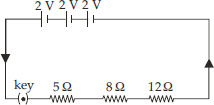Q 13.

Redraw the circuit of question-12, putting in an ammeter to measure the current through the resistors and a voltmeter to measure the potential difference across the 12 Ω resistor. What would be the reading in the ammeter and the voltmeter?

SOLUTION:

The completed circuit diagram is given below: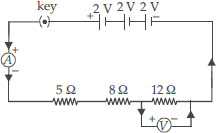For the whole circuit,
Total resistance = 5 Ω + 8 Ω + 12 Ω = 25 Ω
Total applied voltage = 2 V + 2 V + 2 V = 6 V
Then,
current flowing through the resistors,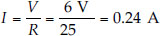So, the ammeter will show a reading of 0.24 A. Voltage across the 12 Ω resistor = IR = 0.24 A × 12 Ω = 2.88 V
So, the voltmeter will show a reading of 2.88 V

Q 14.

Judge the equivalent resistance when the following are connected in parallel:
(A) 1 Ω and 106 Ω,
(B) 1 Ω and 103 Ω and 106 Ω

SOLUTION:

(A) For a parallel combination,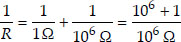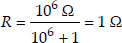(1 is negligible as compared to 106)
(B) For the other parallel combination, we can write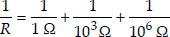= 1 Ω-1 + 0.001 Ω-1 + 0.000001 Ω-1
= 1.001 Ω-1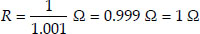Q 15.

An electric lamp of 100 Ω, a toaster of resistance 50 Ω and a water filter of resistance 500 Ω are connected in parallel to a 220 V source. What is the resistance of an electric iron connected to the same source that takes as much current as all three appliances and what is the current through it?

SOLUTION:

Resistance of the electric lamp, R1 = 100 Ω resistance of toaster, R2 = 50 Ω resistance of water filter, R3 = 500 Ω Since R1, R2 and R3 are connected in parallel, their equivalent resistance (Rp) is given by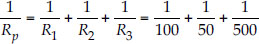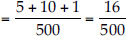or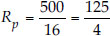Ω
Current through the three appliances, i.e.,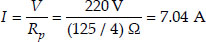Since the electric iron connected to the same source (i.e., 220 V), takes as much current as all three appliance, i.e., I, its resistance is equal to Rp,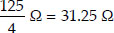Q 16.

What are the advantages of connecting electrical devices in parallel with the battery instead of connecting them in series?

SOLUTION:

(A) When a number of electrical devices are connected in parallel, each device gets the same potential difference as provided by the battery and it keeps on working even if other devices fail. This is not so in case the devices are connected in series because when one device fails, the circuit is broken and all devices stop working.
(B) Parallel circuit is helpful when each device has different resistance and requires different current for its operation as in this case the current divides itself through different devices. This is not so in series circuit where same current flows through all the devices, irrespective of their resistances.

Q 17.

How can three resistors of resistances 2 Ω, 3 Ω and 6 Ω be connected to give a total resistance of
(A) 4 Ω (B) 1 Ω?

SOLUTION:

(A) Following combination will give a total resistance of 4 ΩThus, the resistances of 3 Ω and 6 Ω are connected in parallel and this combination is combined with 2 Ω resistance in series.
(B) By connecting all the three resistances in parallel.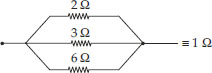Q 18.

What is (A) the highest, (B) the lowest total resistance that can be secured by combinations of four coils of resistances 4 Ω, 8 Ω, 12 Ω, 24 Ω?

SOLUTION:

(A) Highest resistance = R1 + R2 + R3 + R4
= 4 Ω + 8 Ω + 12 Ω + 24 Ω = 48 Ω
(B) If R is the lowest resistance, Then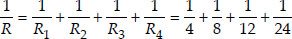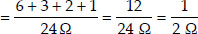So, the lowest resistance of the combination = 2 Ω

Q 19.

Why does the cord of an electric heater not glow while the heating element does?

SOLUTION:

The cord of an electric heater is made of thick copper wire and has much lower resistance than its element. For the same current (I) flowing through the cord and the element, heat produced (I2Rt) in the element is much more than produced in the cord. Consequently, the element becomes very hot and glows whereas the cord does not become hot and as such does not glow.

Q 20.

Compute the heat generated while transferring 96000 coulombs of charge in one hour through a potential difference of 50 V.

SOLUTION:

Here, Q = 96000 C, t = 1 h = 60 × 60 = 3600 s, V = 50 V
Heat produced, W = QV = 96000 C × 50 V = 48 × 105 J.

Q 21.

An electric iron of resistance 20 Ω takes a current of 5 A. Calculate the heat generated in 30 s.

SOLUTION:

Resistance, R = 20 Ω
Current, I = 5 A
Time, t = 30 s
Heat generated = I2Rt = (5 A)2 × 20 Ω × 30 s = 25 × 20 × 30 J = 15000 J = 15 kJ

Q 22.

What determines the rate at which energy is delivered by a current?

SOLUTION:

Rate at which energy is delivered by a current is called electric power.
Electric power is determined by
(A) The potential difference across the conductor in volts
(B) The current in amperes.

Q 23.

An electric motor takes 5 A from a 220 V line. Determine the power and energy consumed in 2 h.

SOLUTION:

Here, I = 5 A, V = 220 V, t = 2 h = 2 × 60 × 60 = 7200 s
Power, P = VI = 220 × 5 = 1100 W
Energy consumed, W
= VI t = 220 × 5 × 7200 = 7920000 J

Q 24.

A piece of wire of resistance R is cut into five equal parts. These parts are then connected in parallel. If the equivalent resistance of this combination is R, then the ratio R/R’
(A) 1/25
(B) 1/5
(C) 5
(D) 25

SOLUTION:

Resistance of each one of the five parts =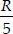Resistance of five parts connected in parallel is given by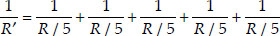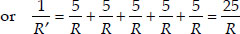or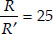Thus, options (D) is the correct answer.

Q 25.

Which of the following terms does not represent electrical power in a circuit?
(A) I2R
(B) IR2
(C) VI
(D) V2/R

SOLUTION:

Electrical power, P = VI = (IR)I
= I2R = V2/R
Obviously, IR2 does not represent electrical power in a circuit.
Thus, option (B) is the correct answer.

Q 26.

An electric bulb is rated 220 V and 100 W. When it is operated on 110 V, the power consumed will be
(A) 100 W
(B) 75 W
(C) 50 W
(D) 25 W

SOLUTION:

Resistance of the bulb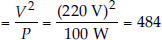Then,
Power consumed at 110 V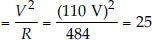Thus, option (D) is the correct answer

Q 27.

Two conducting wires of the same material and of equal lengths and equal diameters are first connected in series and then in parallel in an electric circuit. The ratio of the heat produced in series and parallel combinations would be
(A) 1 : 2
(B) 2 : 1
(C) 1 : 4
(D) 4 : 1

SOLUTION:

Since both the wires are made of the same material and have equal lengths and equal diameters, these have the same resistance. Let it be R.
When connected in series, their equivalent resistance is given by
Rs = R + R = 2R
When connected in parallel, their equivalent resistance is given by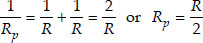Further, electrical power is given by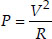Power or heat produced in series,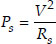Power or heat produced in parallel,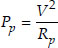Thus,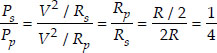or Ps : Pp = 1 = 4
Thus, option (C) is the correct answer.

Q 28.

How is voltmeter connected in the circuit to measure potential difference between two points?

SOLUTION:

A voltmeter is always connected in parallel across the points between which the potential difference is to be determined.

Q 29.

A copper wire has a diameter of 0.5 mm and a resistivity of 1.6 × 10–6 ohm cm. How much of this wire would be required to make a 10 ohm coil? How much does the resistance change if the diameter is doubled?

SOLUTION:

Given diameter of the wire, D = 0.5 × 10–3 m
resistivity of copper, ρ = 1.6 × 10–6 ohm cm
= 1.6 × 10–8 ohm m
required resistance, R = 10 ohm
As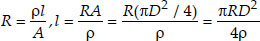[∵ A = πr2 = π(D/2)2 = πD2/4]
or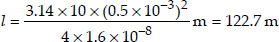Since,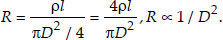When D is doubled, R becomes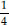times.

Q 30.

The values of current, I, flowing in a given resistor for the corresponding values of potential difference, V, across the resistor are given here :
I (ampere) 0.5 1.0 2.0 3.0 4.0
V (volt) 1.6 3.4 6.7 10.2 13.2
Plot a graph between V and I and calculate the resistance of the resistor.

SOLUTION:

The VI graph is as shown in figure.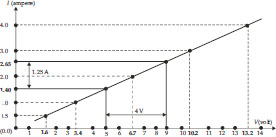For V = 4 V (i.e., 9 V – 5 V), I = 1.25 A (i.e., 2.65 A – 1.40 A).
Therefore,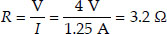The value of R obtained from the graph depends upon the accuracy with which the graph is plotted.

Q 31.

When a 12 V battery is connected across an unknown resistor, there is a current of 2.5 mA in the circuit. Find the value of the resistance of the resistor.

SOLUTION:

Here, V = 12 V, I = 2.5 mA = 2.5 10–3 A
Resistance of the resistor,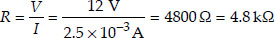Q 32.

A battery of 9 V is connected in series with resistors of 0.2 Ω, 0.3 Ω, 0.4 Ω, 0.5 Ω and
12 Ω. How much current would flow through the 12 Ω resistor?

SOLUTION:

Since all the resistors are in series, equivalent resistance,
Rs = 0.2 Ω + 0.3 Ω + 0.4 Ω + 0.5 Ω + 12 Ω
= 13.4 Ω
Current through the circuit,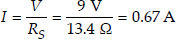In series, same current (I) flows through all the resistors. Thus, current flowing through 12 Ω resistor = 0.67 A

Q 33.

How many 176 Ω resistors (in parallel) are required to carry 5 A on a 220 V line?

SOLUTION:

Let there be n resistors in parallel. Then the equivalent resistance is given by,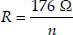From Ohm’s law,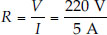So,or n = 4
So, four resistors of 176 Ω each are required.

Q 34.

Show how you would connect three resistors, each of resistance 6 Ω, so that the combination has a resistance of (A) 9 Ω, (B) 4 Ω.

SOLUTION:

(A) The resistance of the combination is higher than each of the resistances. We can obtain 9 Ω by coupling 6 Ω and 3 Ω in series. A parallel combination of two 6 Ω resistors is equivalent to 3 Ω. So, to obtain 9 Ω circuit, the combination (A) given alongside is possible.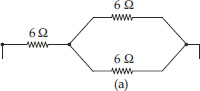(B) To obtain a combination of 4 Ω, we have to connect two 6 Ω resistors in series and then connect the third 6 Ω resistor in parallel of the series combination as shown in the figure (B).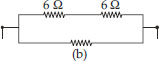Q 35.

Several electric bulbs designed to be used on a 220 V electric supply line, are rated 10 W. How many lamps can be connected in parallel with each other across the two wires of 220 V line if the maximum allowable current is 5A?

SOLUTION:

In a parallel combination, each bulb has the voltage equal to that of the main line, and the sum of the currents drawn by each bulb would be equal to the allowable current.
From the given data,
Current flowing through each bulb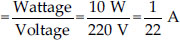Total maximum allowed current = 5 A
No. of bulbs which can be connected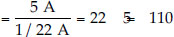Q 36.

A hot plate of an electric oven connected to a 220 V line has two resistance coils A and B, each of 24 Ω resistance, which may be used separately, in series, or in parallel. What are the currents in the three cases?

SOLUTION:

Here, potential difference, V = 220 V resistance of each coil, r = 24 Ω
(i) When each of the coils A or B is connected separately, current through each coil, i.e.,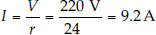(ii) When coils A and B are connected in series, equivalent resistance in the circuit,
Rs = r + r = 2r = 48 Ω
Current through the series combination,
i.e.,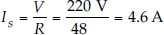(iii) When the coils A and B are connected in parallel, equivalent resistance in the circuit,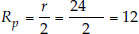Current through the parallel combination,
i.e.,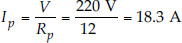Q 37.

Compare the power used in the 2 Ω resistor in each of the following circuits :
(i) a 6 V battery in series with 1 Ω and 2 Ω resistors, and
(ii) a 4 V battery in parallel with 12 Ω and 2 Ω resistors.

SOLUTION:

(i) Since 6 V battery is in series with 1 Ω and 2 Ω resistors, current in the circuit,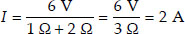Power used in 2 Ω resistor,
P1 = I2R = (2A)2 × 2 Ω = 8 Ω
(ii) Since 4 V battery is in parallel with
12 Ω and 2 Ω resistors, potential difference across 2 Ω resistors, V = 4 V.
Power used in 2 Ω resistor,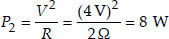Clearly,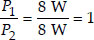Q 38.

Two lamps, one rated 100 W at 220 V, and the other 60 W at 220 V, are connected in parallel to electric mains supply.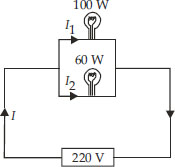What current is drawn from the line if the supply voltage is 220 V?

SOLUTION:

Since both the bulbs are connected in parallel and to a 220 V supply, the voltage across each bulb is 220 V. Then
Current drawn by 100 W bulb,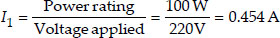Current drawn by 60 W bulb,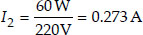Total current drawn from the supply line,
I = I1 + I2 = 0.954 A + 0.273 A = 0.73 A

Q 39.

Which uses more energy, a 250 W TV set in 1 h or a 1200 W toaster in 10 minutes?

SOLUTION:

Energy consumed by TV set = 250 W × 1 h
= 250 J s–1 × 60 × 60 s = 900, 000 J
Energy consumed by toaster
= 1200 W × 10 min
= 1200 J s–1 × 10 × 60 s = 720, 000 J
Thus, the TV set will use more energy.

Q 40.

An electric heater of resistance 8 Ω draws 15 A from the service mains for 2 hours. Calculate the rate at which heat is developed in the heater.

SOLUTION:

Resistance, R = 8 Ω, Current, I = 15 A,
Time, t = 2 h
Rate of generation of heat = I2 × R
= (15A)2 × 8 Ω = 15 × 15 × 8 W = 1800 W

Q 41.

Explain the following :
(A) Why is tungsten used almost exclusively for filament of electric lamps?
(B) Why are the conductors of electric heating devices, such as toasters and electric ions, made of an alloy rather than a pure metal?
(C) Why is the series arrangement not used for domestic circuits?
(D) How does the resistance of a wire vary with its area of cross-section.
(E) Why are copper and aluminium wires usually employed for electricity transmission.

SOLUTION:

(A) Tungsten has a high melting point (3380°C) and becomes incandescent (i.e., emits light at a high temperature) at 2400 K.
(B) The resistivity of an alloy is generally higher than that of pure metals of which it is made of.
(C) In series arrangement, if any one of the applicances fails or is switched off, all the other applicances stop working because the same current is passing through all the appliances.
(D) The resistance of a wire (R) varies inversely as its cross-sectional area (A) as
R ∝ 1/A.
(E) Copper and aluminium wires possess low resistivity and as such are generally used for electricity transmission.